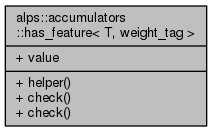ALPSCore reference
alps::accumulators::has_feature< T, weight_tag > Struct Template Reference

#include <weight.hpp>

Collaboration diagram for alps::accumulators::has_feature< T, weight_tag >:## Public Types

typedef std::integral_constant< bool, sizeof(char)==sizeof(check< T >0))> type

## Static Public Member Functions

template<typename R , typename C >
static char helper (R(C::*)() const)

template<typename C >
static char check (std::integral_constant< std::size_t, sizeof(helper(&C::owns_weight))> *)

template<typename C >
static double check (...)

## Static Public Attributes

static constexpr bool value = type::value

## Detailed Description

### template<typename T> struct alps::accumulators::has_feature< T, weight_tag >

Definition at line 31 of file weight.hpp.

## Member Typedef Documentation

template<typename T >
 typedef std::integral_constant0))> alps::accumulators::has_feature< T, weight_tag >::type

Definition at line 35 of file weight.hpp.

## Member Function Documentation

template<typename T >
template<typename C >
 static char alps::accumulators::has_feature< T, weight_tag >::check ( std::integral_constant< std::size_t, sizeof(helper(&C::owns_weight))> * )
static
template<typename T >
template<typename C >
 static double alps::accumulators::has_feature< T, weight_tag >::check ( ... )
static
template<typename T >
template<typename R , typename C >
 static char alps::accumulators::has_feature< T, weight_tag >::helper ( R(C::*)() const )
static

## Member Data Documentation

template<typename T >
 constexpr bool alps::accumulators::has_feature< T, weight_tag >::value = type::value
static

Definition at line 36 of file weight.hpp.

The documentation for this struct was generated from the following file: